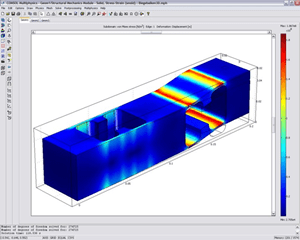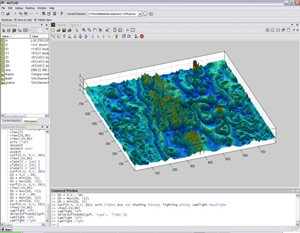# Numerical Simulation, Data Analysis & Software Development

### High performance computing cluster

computer simulations and other complex numerical tasks with high demand on computational power, primarily parallelised problems or large-scale parameter studies.

using the workload manager SLURM, computation tasks can be automatically assigned to free processor resources; using MPI functionality, calculations can be distributed to several nodes and executed in parallel on more than 1000 processor cores

IBM iDataPlex Cluster consisting of 84 nodes with 16 processor cores and 64 GB RAM per node (total of 1344 cores and 5376 GB RAM); infiniband network; total computing power: approx. 25 Tflops; operating system: LinuxVORLAUFER Georg

### Mathematica Software

symbolic mathVORLAUFER Georg

### Ansys (finite element analysis software)

Solution of linear and nonlinear problems from structural mechanics, fluid mechanics, acoustics, thermodynamics, piezoelectricity, electromagnetism as well as combined problems (multiphysics).

Numerical solution of a complex system of differential equations.To solve a problem, the FEM subdivides a large system into smaller, simpler parts that are called finite elements. This is achieved by a particular space discretization in the space dimensions, which is implemented by the construction of a mesh of the object: the numerical domain for the solution, which has a finite number of points. The finite element method formulation of a boundary value problem finally results in a system of algebraic equations. The method approximates the unknown function over the domain. The simple equations that model these finite elements are then assembled into a larger system of equations that models the entire problem. The FEM then uses variational methods from the calculus of variations to approximate a solution by minimizing an associated error function.

Strength and deformation investigation of solids with geometrically complex shape as well as investigation of scientific problems in the field of fluid mechanics, thermodynamics and acoustics.KRENN Stefan

### COMSOL Multiphysics software package

modelling/simulation of physical processes (e.g., structural mechanics, thermal effects, eigenfrequency analysis, fluid-structure interaction, coupling of electro-dynamic, thermal, and mechanical effects, contact mechanics)

by means of a graphical user interface, real two- and three-dimensional geometries can be modelled and mesheds; physical phenomena can be freely defined and combined on these geometriess; for the most common physical processes predefined equations are provided; boundary conditions and material properties have to be defined; COMSOL provides solvers for linear/non-linear stationary analyses

Windows/Linux software in the latest version; Comsol Multiphysics base package with various toolboxes (add-ons); also runs on the clusterKRENN Stefan

### Matlab software package

complex numeric computational tasks (e.g. data analysis and visualisation, optimisation tasks, image analysis, development of algorithms)

by means of MATLABs powerful library of numerical algorithms (e.g. for the inversion of matrices, fast fourier transform, spectral analysis, solution of nonlinear systems of equations), complex computational tasks can be realised efficiently; an extensive toolbox for the generation of graphical user interfaces and for high qualitity visualisation of computation results is avaliable; complex calculation processes can be automated by means of scripts#### Contact

KRENN Stefan

modelling and simulation of dynamic systems with a small number of degrees of freedom (e.g. single or multiple mass oscillator, stick-slip motion, control systems)

generation of abstract models of dynamic systems by means of block diagrams (graphical user interface); simulation of systems by means of numerical solution of the equations of motion (coupled linear or non-linear ordinary differential equations)KRENN Stefan

### Molecular dynamic software

open source molecular dynamics simulation softwareKRENN Stefan

### Vienna Ab-initio Software Package

simulation softwareKRENN Stefan

### TriboSoft V1 software package

automated test stand control and data acquisition software

Software package has been developed by AC²T based on LabView; it is designed using a modular (plugin) architecture; with this software a flexible design of test procedures and data acquisition tasks can be realized for tribometer and test rigs